﻿ Linde-Hampson Anti-Machine: Self-heated Compressed Van-Der-Waals Gas as an Energy Carrier for Pneumatic Vehicles

### Linde-Hampson Anti-Machine: Self-heated Compressed Van-Der-Waals Gas as an Energy Carrier for Pneuma...

E.Ya. GlushkoOPEN ACCESSPEER-REVIEWED

## Linde-Hampson Anti-Machine: Self-heated Compressed Van-Der-Waals Gas as an Energy Carrier for Pneumatic Vehicles

Institute of Semiconductor Physics of the NAS of Ukraine, Nauki Ave, Kiev, Ukraine

### Abstract

In this paper, the problem of increasing the energy capacity of compressed air used as a mobile carrier of energy is considered. An idea of preliminary Joule-Thomson self-heating of the compressed air with the following heat redistribution to obtain the maximal work at maximal power is considered. A simple circle combination of a heat exchanger and a throttle device-Linde-Hampson anti-machine - is analyzed. Calculations performed in the framework of modified van der Waals gas model show essential increasing of accumulated specific energy of compressed air fuel to the range 0.5-1.0 MJ/kg which is comparable with electrochemical fuel energy. A concept of compressed air fuel production is discussed.

### At a glance: Figures

123
Prev Next

• Glushko, E.Ya.. "Linde-Hampson Anti-Machine: Self-heated Compressed Van-Der-Waals Gas as an Energy Carrier for Pneumatic Vehicles." American Journal of Energy Research 1.3 (2013): 59-67.
• Glushko, E. (2013). Linde-Hampson Anti-Machine: Self-heated Compressed Van-Der-Waals Gas as an Energy Carrier for Pneumatic Vehicles. American Journal of Energy Research, 1(3), 59-67.
• Glushko, E.Ya.. "Linde-Hampson Anti-Machine: Self-heated Compressed Van-Der-Waals Gas as an Energy Carrier for Pneumatic Vehicles." American Journal of Energy Research 1, no. 3 (2013): 59-67.

 Import into BibTeX Import into EndNote Import into RefMan Import into RefWorks

### 1. Introduction

The compressed gases have found wide usage in various technical applications. In particular, compressed air remains irreplaceable in aviation and cosmonautics due to its good ecological properties and other features. Also, compressed air is used as a mobile carrier of energy in starters of heavy diesel engines and medicine. The ability of compressed air-to be a mobile carrier of energy, is in centre of our study. The question is how much mechanical work may be drawn out of this carrier? Recently an appreciable progress has been achieved in competitive transportation means which use the pneumatic fuel . Nevertheless, relatively low energy efficiency of compressed air of the order of 0.1-0.2 MJ of mechanical energy per 1 kg [2, 3] seriously weakens its position as an effective carrier of energy for transport vehicles in comparison, for example, with electrochemical vehicles where energy capacities have magnitudes more than 0.5 MJ/kg .

Therefore the investigation of ways to increase energy capacity of compressed air up to magnitudes 0.5-1.0 MJ/kg and even more at working pressures 30-90 bar is very important. In this work, so-called negative Joule-Thomson effect is proposed to use for the van der Waals gas self-heating . At relatively high pressures the throttling process is accompanied by temperature increasing due to negative work. Like the well-known Linde machine which uses a gas self-cooling during the throttling inside a heat exchanger at the condition of positive Joule-Thomson effect (dP/dT>0), an analog taken in area dP/dT<0 should cause self-heating of the gas. The latter phenomenon is not jet investigated properly in literature and our study discusses possible use of negative Joule-Thomson effect to create a novel mobile carrier of energy. Also general aspects of Linde’s anti-machine are considered from the point of view of increasing the energy capacity of compressed air fuel (CAF) to region of the order of 0.5-1.0 MJ/kg and more.

### 2. Energy and Enthalpy Maps of a Real Gas

We consider compressed air as the carrier of energy. In a modified van der Waals approximation 1 kg of dense gaseous medium taken at pressure P and temperature T may be described by the phenomenological equation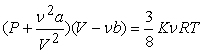(1)

where v is number of moles in 1 kg, K is modification constant (8/3 in classic case, 3.42 for N2), V, volume, R, universal gaseous constant, a and b are the Van der Waals constants. The internal energy is expressed by equation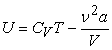(2)

where Cv is the specific heat capacity of the gas at the constant-volume process. Further we will refer to one more thermodynamic function of state, the enthalpy H = U + PV. This function plays an important role in our further consideration of the compressed air ability to store energy. It expresses the heat properties of a working body - dense gas, in a thermodynamic process. In the case a dense gas is throttled through a narrow channel or a system of narrow channels the enthalpy function H remains constant. The throttling or Joule-Thomson process is accompanied by work the gas does on its surrounding. Therefore the reliefs of intrinsic energy and enthalpy in PT-space allow to analyze the ways to extract energy accumulated by compressed gas for its following transformation into mechanical work. We define the mechanical work W as an integral along the corresponding line of the process. At that the negative magnitude of W corresponds to the case when the surrounding medium performs a work on the gas. It is of interest that negative work is peculiar to Joule-Thomson process [5, 6]. To evaluate the work in this case one should take the initial and final points of the integration curve at the ends of the chosen isenthalpic line, therefore W = (PV)i-(PV)f. As a result we have increasing internal energy U if the Joule-Thomson process is of negative kind. In this work, we will use the constants a and b averaged by the Kopp-Neumann rule for air mix of nitrogen and oxygen: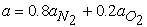,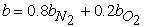.

As to the Joule-Thomson process, the phase diagram in РТ plane is divided on two connected regions of positive and negative coefficient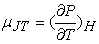. For an arbitrary non-classic van der Waals gas when K≠8/3 the demand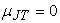leads to the following expression for inversion curve Tμ = 0(P) [5, 6]: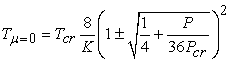(3)

where Pcr and Tcr are critical pressure and temperature. In Figure 1a, calculated constant density lines 10, 20, 30710 kg/m3 (right and upper axis) for compressed are plotted. In the chosen model of Van der Waals gas the isodenses are direct lines. As it can be seen from figure, the constant density lines corresponding to magnitudes in a wide range beginning with 50 and up to 700 kg/m3 lay close one to another in vicinity of the critical point Ĉ what explains the extremely weak compressibility and strong fluctuations in the critical point. Shown are line of vapour-liquid equilibrium ĈS connecting the critical point Ĉ and triple point S as well as the line of solid-liquid equilibrium SS’. Since our purpose is to study the energy stored in compressed gas we consider several important thermodynamic states in the PT plane. The atmosphere point A(1, 300) also may be treated as the final state of adiabatic expansion of hot compressed air inside the engine beginning with the initial point B(96.3, 1400) for K = 3.42 and B(223.5, 1400) at K = 8/3. If the final point of adiabatic expansion process is taken A(1, 350) then initial point is located at B(19.6, 1400) for K = 3.42 and B(130.8, 1400) at K = 8/3. The intermediate point B’(300, 1380) is a supposed final point of the Joule-Thomson process accompanied by heat extracting. In this work, we consider the case when all the process begins with state C(300, 700). The model contains an unstable two-phase zone (dP/dV)T>0, which is marked with dark gray color in the left corner of the diagram. The bold line connecting point C and the unstable two phase zone corresponds to isochoric heating of liquid air at average density 415 kg/m3. The critical and triple points are marked with Ĉ and S, correspondingly.

There are many studies devoted to inversion curves for various dense gases [7, 8, 9]. A good approximation to the experimental Joule-Thomson inversion curve for a few gases, including nitrogen, was found in  (curve 3) whereas the classic (K = 8/3) and modified (K = 3.42) versions of the Van der Waals equation give curves 1 and 2 in Figure 1a, correspondingly. Our estimations show that curve 2 becomes totally close to the experimental curve 3 if the transformation Pcr à 1.3Pcr is made in the framework of chosen van der Waals model.

Figure 1. (Color online) The Van der Waals model. (a) Iso-density lines for the classic van der Waals gas: 10, 20, 30… 710 kg/m3-labelled at right and upper axes, A, B, B’, C, chosen special points on the PT plane: A(1,300), B(96.3, 1400), B’(300, 1380), C(700, 300); 1, classic Joule-Thomson inversion curve (K = 8/3) , 2, Joule-Thomson inversion curve at K = 3.42 (air), 3, approximate Joule-Thomson inversion curve built by , Ĉ, critical point, S, triple point, SS’, line of solid-liquid equilibrium, ĈS, line of vapour-liquid equilibrium; dark gray rectangular, two-phase unstable zone; bold line connecting point C and the unstable zone corresponds to isochoric heating of liquid air. (b) Calculated P-T diagram for internal energy of compressed air. Parameter K = 3.42; isoenergetic curves: Us = 0.05·(s-1), s = 1, 2.15, max{U}<1.1 MJ/kg; UA = 0.217 MJ/kg, UB = 1.011 MJ/kg, special energy of prepared fuel at the initial point of adiabatic expansion, UB’ = 0.989 MJ/kg, special energy of preliminary prepared fuel, UC = 0.148 MJ/kg, special energy of fuel in tank.

In Figure 1b, the diagram of internal energy calculated by finite difference method for one kg of compressed air in PT plane is shown. The U(P,T) diagram ranges pressures up to 800 bar and temperatures up to 1500 K. The map of the internal energy dependence is described by 16 isoenergetic curves numbered beginning with zero specific energy up to 1.05 MJ/kg with a difference in approximately 0.05 MJ/kg: Us = -0.05 + 0.05·s, s = 1,2..22. The parameter K in the modified van der Waals equation of state (1) to be 3.42. A motivation to choose the working point A, B, B’, C follows from Figure 1b, where the system of isoenergetic curves covers the actual range of parameters: A represents the final point of the adiabatic process (UA = 0.217 MJ/kg), B is the initial point of the adiabatic process (UB = 1.011 MJ/kg). Below, the role of special point B’ as an intermediate state of preliminary prepared compressed air fuel (UB = 0.989 MJ/kg) will be explained. At last, the thermodynamic state of compressed air in point C is chosen as an initial state of fuel before the reinforced process is started (UC = 0.148 MJ/kg). The diagram of internal energy is convenient to determine the possible mechanical work, which may be picked out the compressed air fuel in an adiabatic process. The difference between points А and В(1400 K, 1.011 MJ/kg) equals to 0.794 MJ/kg, what is of interest to consider air as a fuel. The jump of the internal energy between points A and C is about 0.069 MJ/kg whereas the needed minimal isothermic work to produce compressed air in point C is about 0.692 MJ/kg. The calculated amount of extracted heat in the isothermic process Aà C is about 0.383 MJ/kg.

Figure 2. (Color online) Calculated air enthalpy P-T diagram. Isenthalpic curves: H = 0.95·s MJ/kg, s = 1,2…16; A, B, B’, C, chosen special points (Figure 1); a, Joule-Thomson inversion curve at K = 3.42, b, approximate Joule-Thomson inversion curve built by (Figure 1, curves 2, 3); lines c and d note the Joule-Thomson process possible pressure limits 300bar and 700 bar

In Figure 2, the calculated by finite differences diagram of enthalpy for one kg of compressed air in PT plane is shown where isenthalpic curves s = 1,2…16 correspond to formula H = 0.95·s MJ/kg. The arc a is plotted by the Joule-Thomson inversion curve (3) taken at K = 3.42 whereas the arc b is the approximate Joule-Thomson inversion curve built by data  (also Figure 1, curve 3). Both these curves confine the area of positive inclination of the enthalpy contour lines (positive Joule-Thomson effect) within intervals of pressures P<9Pcr ≈ 329 bar and temperatures 77≥Т≤697 K for the modified Van der Waals gas at K = 3.42. The throttling process taken inside these arcs leads to decreasing of temperature. The outside area may be used to increase the temperature in the process of throttling. The polynomial approximation to the experimental Joule-Thomson inversion curve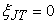was found in  for gaseous N2, Ar, and other gases (Figure 2, curve b, Figure 1a, curve 3). The lower and upper lines c and d depict possible pressure limits 700 bar and 300 bar in a throttling process. The chosen special points A, B, B’, C, were discussed above.

Note that two ways to produce a kg of compressed air fuel i.e., to reach the position C on the pressure-temperature plane, exist. The first way is represented by an isochoric transfer of one kg of liquid air taken at 1 bar into the state C shown in Figure 1a by the bold line. This process needs an amount of heat about 0.15 MJ that should be added to energy expended during the liquefying process. To avoid wasting of energy one more demand should be fulfilled-the initial gas-liquid mixture density must exceed critical density 331 kg/m3 of our van der Waals model with accepted Kopp-Neumann rule. As follows from Figure 1a, the shown isochoric line corresponds to density 415 kg/m3.

Figure 3. (Color online) Calculated specific energy and density of the van-der-Waals model of air at K = 3.42. 1, isothermal compression work (PA = 1bar) vs final pressure PC (left axis). 2, fuel density measured in g/cm3(right axis)

Further we will consider the second way which is based on direct compressing beginning with rarefied state of air at atmospheric pressure and room temperature. Since states A and C are taken at a coinciding temperature, the minimal work at the path Aà C may be reached in the isothermal process. In Figure 3, shown are the dependences of energy expense and density of final state of compressed air on the final state pressure PC by an isothermal process in interval ranged from initial pressure 1 bar to the final 1000 bar at 300 К (Figure 1, Figure 2, point A). The calculation gives for the chosen initial thermodynamic parameters of fuel PC = 700 bar and TC = 300 K the energy of production W = 0.692(0.5215 at k = 8/3) MJ/kg and fuel density ρ = 413 kg/m3. It should be emphasized, that relative increasing of density at PC <300 bar (curve 2) is not significant whereas the CAF energy capacity (curve 1) seriously hoicks at small pressures and then transfers into a plateau: W(300) = 0.621 MJ/kg, W(1000) = 0.718 MJ/kg.

In this work, a way to reinforce the real gas energy capacity using the negative Joule-Thomson effect is considered. The energy capacity i.e., work that a gas may perform in an adiabatic expansion process, depends on the location of point B on the PT plane. By technical conditions, the pressure should become atmospheric at the end of the process whereas the final temperature in general case not coincides with ТC. Moreover, the less is temperature TA, the more is useful mechanical work obtained in the process. The bigger magnitudes of PB and PB correspond to bigger energy capacity of the compressed air. Due to the negative Joule-Thomson process performed in area limited by lines c and d in Figure 2 the compressed gas transfers from the point С to point B’ at the PT plane and then to point B. From this point of view, the required magnitudes PC begin with pressures of the order of 400 bar and higher in correspondence with curves a and b in Figure 2. It worth to note, that a simple isenthalpic process leads to a weak growth of temperature at these conditions. In Sections 3 and 4, nevertheless, we will show how the use of a heat exchanging procedure helps to extend the range of final temperatures TB’.

The adiabatic work W(TA, TB) done by one kg of a dense gas may be found both immediately from the P-T diagram for internal energy of compressed air shown in Figure 1b and as a process function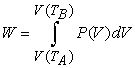(4)

The surface W(TA, TB) calculated in axes TA, TB is plotted in Figure 4. For the chosen interval of temperatures, the surface W(TA, TB) is practically plain. The maximal work 0.77734 MJ/kg corresponds to point W2(120, 1300), whereas the minimal zeroth one corresponds to nearest right point (300К, 300К). The most interesting for technical aims interval of pressures from 30 bar to 90 bar is highlighted by light gray. A conclusion can be made from the behavior of the highlighted band that to obtain specific work more than 0.6 MJ/kg one should use the regimes with outlet temperatures TA more than 300 K. The pressure of shown points W(TA, TB) in the highlighted band increases along the direction of gradient, whereas magnitudes of the done work decrease along the band from left to right. Therefore, the most acceptable point of area TB≤1300 K should be acknowledged a point with maximal work in the highlighted band. Our calculation gives for this region the point W(300,1079.4) with pressure PB = 90 bar and potential work W = 0.559 MJ/kg. The nearest left point of the surface W(300,1300) = 0.7165 MJ/kg has PB = 172.5 bar. The most remote left point of the surface W2 corresponds to PB = 4.086 Kbar that exhibits relatively strong pressure increasing along the surface gradient.

The adiabatic work surface shown in Figure 4 being close to a plane may be approximated by the equation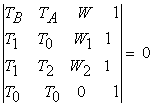(5)

where T0 = 300K, T1 = 1300K , T2 = 120K, W(T1, T0) = W1 = 0.7165 MJ/kg, W(T1, T2) = W2 = 0.84 MJ/kg. The expression (5) gives a very good approximation for nearest low temperature part of the adiabatic work surface at chosen parameters, in the middle of the shown surface in point W(240, 650) the accuracy is about 0.6%, whereas the most remote high temperature part of the surface gives value W2 = 0.84 MJ/kg instead of the exact one 0.77734 MJ/kg (15%) and for the low temperature point W3 = 0.12351 MJ/kg instead of the exact magnitude of potential work 0.12595 MJ/kg (2%). As the matter of fact, the considered way gives minimal energy to produce one kg of compressed air fuel in point C(700, 300) about 0,693 MJ, at the same time the difference UA-UC ~ 0,069 MJ may be transformed into heat and returned. The same surface taken at K = 3.42 is more attractive in applied sense: though the both reference points W1 and W2 are close to previous ones, the technically important band of pressures 30<P<90 bar now begins from the very left corner of the surface, and it includes point W1 = 0.721 MJ/kg. Therefore an opportunity arises to operate by work magnitudes more than 0.5 MJ/kg at temperatures TA ranged from 200 K to 300 K.

Figure 4. (Color online) Calculated surface of specific work W depended on the adiabatic process limit temperatures TA, TB. The transverse dotted lines s = 1, 2…7 correspond to energies Ws = 0.1·s MJ/kg; K = 8/3

The relatively high pressure of the considered carrier of energy in state С allow the compressed gas to be transmitted into a state with higher internal energy which in turn may be converted into mechanical work.

### 3. Linde-Hampson’s Anti-Machine: to Reinforce the Compressed Air Fuel

Here we consider a way to transform the compressed gas from a state most convenient for storing in a tank to a state suitable to produce maximal work at maximal power using the throttling process. During the Joule-Thomson process, the working body at point C(300, 700) may be transmitted into an arbitrary state B’ lying at the corresponding isenthalpic curve (see Figure 2). Motion along the isenthalpic curve beginning with point C at 700 bar to an appropriate point B’ at 300 bar causes the temperature shift about 23.4 К at K = 8/3 and 17.1 К at K = 3.42. Due to the negative work the system does under the van der Waals gas, the internal energy increases to about 0.046 MJ/kg at K = 8/3 and 0.042 К at K = 3.42. With the help of a heat exchanger which redistributes the heat between the input and output of the throttle the working body state may be transmitted into any point B’ of the Р-Т plane inside the pressure interval P<PC. In Figure 5a, the Linde-Hampson anti-machine design is schematically shown. It includes the heat exchanger and throttling device connected so that output of the throttling device creates the hot circle of exchanger whereas the cold exchanger branch is the input of the throttling device. The axis X shows chosen direction of increasing for gas coordinate x (L≥ x ≥0) both in the input circle before the throttle 2 and output circle after throttling. Just when the heat exchanger begins to act, the leg of isenthalpic curve СB’ begins to shift in side of a higher temperature. Therefore the energy gain may reach 1 MJ/kg and more. The time interval of this regime establishing will be evaluated below, it may reach seconds or dozens of seconds depending on the quality of the heat exchanger. It is worth noting that the energy capacity of the considered working body may exceed the minimal work spent initially to produce 1 kg of the compressed air fuel (point С). On the one side, the exceedence arises due to increasing of internal energy of a real gas in considered Joule-Thomson process and, on the other side, one more way exists of energy capacity rising - to chose the outlet temperature ТА lower than fuel temperature ТС. Doing so, one can formally reach the fuel performance factor bigger than 100%. One more circumstance is of interest: if the temperature ТB’ is high enough, what is the nature of the additional energy reached in a throttle procedure accompanied by the heat exchange? This and other questions like principal restrictions of gain and maximal magnitudes at given initial parameters depend on taken model and needs more detailed investigation.

### 4. An Introduction to the Theory of Joule-Thomson Heat Exchanger

In contrary to the well known Joule-Thomson cooler or Linde-Hampson machine [10, 11, 12, 13, 14, 15], the proposed anti-machine acts like a heater outside the inversion region which is marked by arcs in Figure 1a and Figure 2. One more difference is manifested in different pressure regimes for heater and refrigerator. The cooling effect arises at relatively low decompressing pressures whereas the Linde-Hampson anti-machine needs a jump of several hundred bar between the input and output chambers of throttling device

As it follows from Figure 2, the heat exchanger increases temperature of compressed gas decreasing simultaneously its pressure to point B’. And the final stage of reinforcing procedure is one more throttling process decreasing pressure to the working pressure magnitude РB, which is also accompanied by a little increasing of temperature when the phase point moves from the position B’ to position B.

In Figure 5a, the Linde-Hampson anti-machine design is schematically shown. It includes the two-loop heat exchanger united with a throttling device. The intake compressed air fuel enters the heat exchanger in point C(PC, TC) and left the system in point B’(PB’, TB’). The first, high pressure, loop is kept at pressure PC and the second one is at pressure PB’. Due to the difference PC PB’ the throttling process performed in area between lines c and d (Figure 2) leads to heating of the flowing gas depending on the heat exchanger parameters L and α. The throttle device 2 divides the high pressure input chamber PC = 700 bar with compressed gas fuel and the low pressure output chamber 1 at PB’ = 300 bar.

The heat exchange processes in the proposed simplified system may be described in time by two equations for temperature distributions T(x) and Ѳ(x) inside the input and output circuits CPC’ and PB’B’ shown in Figure 5a: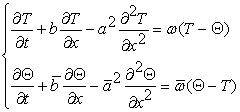(6)

where L≥ x ≥0, the parameters of the equation (6):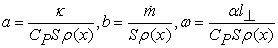(7)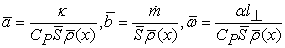(8)

Here κ(x) is thermal conductivity coefficient, α(x) is the heat exchange coefficient measured in W/m2K,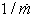is fuel consumption,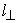is the perimeter of heat contact between cold and hot tubes so that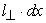gives the corresponding elementary surface of heat contact; S,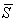are sections of cold and hot tubes inside the heat exchanger. The inhomogeneous gas density distributions are indicated as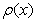and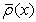. The equations (6) take into consideration the gas movement, thermal diffusion in both branches and heat exchange between hot and cold branches. The exchange of heat between the hot and cold branches of the exchanger is described by the right parts of the system (6). In general the heat exchange processes are essentially depended both on the system design and the dense gas properties [12, 13] therefore we undertake the simplified approach (6) to analyze the principal parameters of the Linde-Hampson anti-machine.

Our estimation show that for a 10 kW power vehicle the average density magnitudes are~0.4 g/cm3,~0.2 g/cm3 and the corresponding mean velocities are of the order of 40 cm/s and 20 cm/s for sections S,which are taken about 1 cm2.

The needed partial solution of equation (6) depends on the initial and boundary conditions.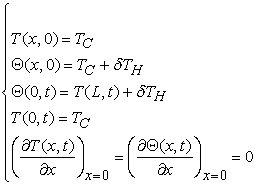(9)

where δTH(t) has been determining at every moment of time as the temperature jump caused by the Joule-Thomson process. To evaluate in general the parameters of the Linde’s anti-machine, further we develop an analytical approach neglecting the nonhomogeneity of coefficients in both equations (6), then after the transfer from temperatures.

The general solution of (6) may be obtained using the discrete Fourier transform in linear space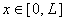of dimension N = 2n + 1. The direct space is determined by coordinates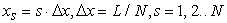(10)

whereas points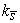of the reciprocal space are chosen as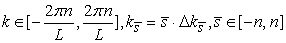(11)

It is easy to find the set of basic functions of the approach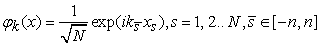(12)

obeying the demands of completeness, orthogonality and normality. As a matter of fact, the parameters in equations (6) like a, b, ω have a nonhomogeneous nature due to the coordinate dependence of density in both branches of the exchanger. To evaluate in general the parameters of the Linde’s anti-machine, further we develop an analytical approach neglecting the nonhomogeneity of coefficients in both equations (6), then after the transfer from temperatures T(x, t), Ѳ(x, t) to Fourier images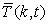,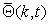the system (6) takes the form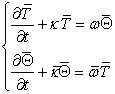(13)

The general solution of (13) found for the constant coefficients κ,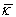, ω and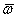gives the time behavior of the Fourier componentsand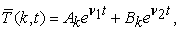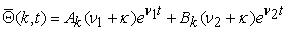(14)

where the roots ν1, ν2 of the characteristic equation have the following view: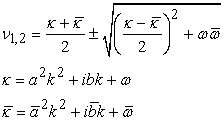(15)

Further we will take into account that the roots νj have real and imaginary parts ξj and ζj , correspondingly (j = 1,2). Then the general solutions for T(x, t) and Ѳ(x, t) may be written in a real view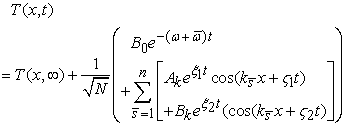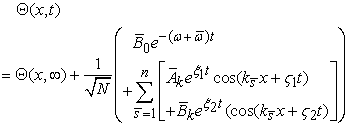(16)

where the real coefficients Ak , Bk which are present in the expansions (16) should be found from the first two boundary equations in (6). The coefficients of expansion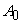,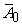have been transformed here in T(x, t) and Ѳ(x, t) taken at t→∞ when the expressions in brackets go to zero. Approximate time of transfer to infinity may be taken as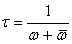(17)
Figure 5. (Color online) (a) Approximate design of the Linde-Hampson anti-machine:1, heat exchanger: inletting cold high pressure gas, 2, throttling device and ambient hot low pressure gas. The fuel intake point, C(PC, TC), heated fuel point, B’(PB’, TB’), PC, pressure of the input loop, PB’ , pressure of outlet loop. (b) Calculated by (6) final (t>>τ) temperature distribution curves T(t, x), Ѳ(t, x): initial, 1, 2 and final, 1’, 2’(cut at T = 1500 K). PC = 700 bar, TC = 300 K, PB’ = 300 bar, TB’ = 1134 K, Tmax(x = 0) = 1937 K, α = 150 W/m2·K, L = 1.6 m, τ = 22.4 s, δTH = TC’- TB’ = 16.13 K’

Figure 5. (Color online) (a) Approximate design of the Linde-Hampson anti-machine:1, heat exchanger: inletting cold high pressure gas, 2, throttling device and ambient hot low pressure gas. The fuel intake point, C(PC, TC), heated fuel point, B’(PB’, TB’), PC, pressure of the input loop, PB’ , pressure of outlet loop. (b) Calculated by (6) final (t>>τ) temperature distribution curves T(t, x), Ѳ(t, x): initial, 1, 2 and final, 1’, 2’(cut at T = 1500 K). PC = 700 bar, TC = 300 K, PB’ = 300 bar, TB’ = 1134 K, Tmax(x = 0) = 1937 K, α = 150 W/m2·K, L = 1.6 m, τ = 22.4 s, δTH = TC’- TB’ = 16.13 K’

In Figure 5b, the initial (t = 0) and final (t>>τ) curves of temperature distribution Ѳ(x, t) and T(x, t) calculated by the equations (6) and solution (16) are plotted. The initial temperature jump δTH (0) = TC - TB’ in (9) was found as 17.1 K, whereas the evaluation gives δTH = TC’- TB’ = 16.13 K for final times t>>τ. The coefficient of heat transfer ‘α’ depends on several factors including the nature of fluids at both sides of the wall, wall surface geometry, fluid velocity and thermal conditions. Different sources give ‘α’ for dense gases at pressures of hundreds bar in a wide interval from hundreds to thousands of W/m2K. Following  we have chosen for our estimating model α = 150 W/m2·K. The calculation shows that at taken heat exchanger parameters and L = 1.6 m the temperature gradient in the high pressure and low pressure circuits is established near 502 K/m through the interval of time τ = 22.4 s. There exists a strong dependence of the final temperature distribution and the inlet temperature Ѳ(∞, L) on the heat exchanger parameters α, S and L. The corresponding combination of these parameters allows one to find a wide spectrum of work regimes. One more circumstance is important that though the negative Joule-Thomson effect at room temperatures has the lowest pressure limit 300 bar (or 400 bar by data ), the process of fuel reinforcing keeps its efficiency up to 100 bar. Therefore, the upper pressure limit, shown by line d in Figure 2, may also be lowered.

In Figure 6, the intake temperature ѲL = Ѳ(L, ∞) dependence in PC ,PBaxes is shown for the model K = 3.42. The most attractive technically region of 800K<ѲL<1200K is highlighted by light gray. The chosen temperature interval corresponds to possible mechanical work inside an interval from 0.5 to 0.8 MJ/kg at pressures not higher than 90 bar according to obtained data of the fuel energy capacitance.

Figure 6. (Color online) Calculated by (13) the intake temperature ѲL = Ѳ(L, ∞) dependence in the PC-PB’ plane. K = 3.42, α = 150 W/m2·K, L = 1.6 m, surface is cut at ѲL = 2500 K; light gray (green online) band corresponds to 800 K< ѲL <1200 K.

To explain the nature of energy reinforcing during the Joule-Thomson process one should take into account the inevitable decreasing of initial pressure РС in tank with time. As the matter of fact, the initially forced gain turns by corresponding loss at final stages of the process when the fuel pressure in a tank is sufficiently decreased. Nevertheless if knowingly stop the process remaining some amount of ballast fuel in the tank, then the proposed way то allows significantly increase the energy capacity of compressed air.

Above the main idea of reinforcing the fuel parameters for a pneumatic thermodynamic engine was considered. Like a steam engine, this thermodynamic engine produces mechanical work due to the energy of compressed gas without using oxidation or any other chemical process.

### 5. General Scheme of CAF Circulation

Note that mentioned above way to prepare the initial state C using an isochoric transfer of one kg of liquid air beginning with 1 bar pressure at density 0.415 g/cm3 (Figure 1a, bold line) needs an amount of heat about 0.15 MJ. It may be taken from the heat exchanger. As a result the working point C due to the correspondingly modified heat exchanger will shift to lower temperatures for approximately 200 K. For example, B(96.3, 1400) à B(61.0, 1200) and specific adiabatic work decreases from 0.793 MJ/kg to 0.649 MJ/kg. Here we remain outside the scope of our study technical questions like pressure keeping systems or ways to transport liquid air to state C in an isochoric process. In Figure 7, shown are six types of pressure regimes in a pneumo-vehicle based on liquid air fuel. The process of fuel reinforcing inside the engine begins from liquid air in a tank (cycle 1), which intakes (cycle 6) into the circuit of high pressure 700 bar in the isochoric process with heating due to the energy transfer from the high temperature cycle 3 to cycle 4. The increasing of temperature during the process 6 needs about 0.15 MJ/kg. Therefore the output temperatures TB and TB’ of cycle 4 should be decreased to approximately 200 К from 1200 K to 1000 K. The latter in turn is the temperature of the fuel intake in the engine cylinder to produce mechanical work. As was mentioned above the intake pressure should be chosen in an approximate interval from 30 to 90 bar. The heat exchanger output 300 bar pressure cycle 3 (curve 2 in Figure 5a) is characterized by the temperature fall from about 2100 K to 1200 K due to heat flows out of the cycle 3. The pre-injection pressure cycle 5 corresponds to state B shown in Figures 1 and Figure 2. In general the parameters of state B should be chosen in technically valuable band of pressures 30-90 bar corresponding the internal combustion and diesel engines. In time when mechanical work is performed the working body state transforms from point B to point A. The cycle 3 to cycle 5 temperature drop 1200à 1000 is caused by a need to supply the isochoric liquid-gas transfer of the cycle 6. Also, cycle 2 is shown in Figure 7 servicing the pneumo-system of the vehicle: doors, windows, windshield viper blade and conditioning.

Figure 7. (Color online) Six cycles of pressure in a pneumo-vehicle. 1, liquid air in tank; 2, servicing pneumo-system; 3, heat exchanger’s output pressure cycle; 4, heat exchanger’s input pressure cycle; 5, pre-injection pressure cycle; 6, fuel heating isochoric cycle

An important point of our study is technical perspective of air as the energy carrier and energy accumulator called to replace gasoline filling stations and oil bases. In a more wide treatment other gases or fluids also may be considered as carriers of mobile energy. Of course, additional amount of energy is needed to prepare compressed gas or steam fuel and new kinds of sources should replace in future the niche of traditional sources. Therefore, the considered conception implies an idea of restructuring in power engineering so that electric energy partially is used in compressors to produce compressed air and partially returns to the power system through a new kind of electric power stations transforming heat which accompanies the compressed air fuel production into electrical energy. This new kind of power stations may be inscribed in existing systems of accumulating power stations. Many unsolved problems exist including the investigation of unknown phase diagrams of gaseous mixtures at high pressures.

Figure 8. (Color online) A concept of oxygen depleted air circulation: Industry-Fuel Station-Vehicle-Atmosphere.

A preliminary concept of energy circulation in a world of compressed air fuel is proposed in Figure 8. The process is started from industry of liquid air consuming electric energy at off-peak stages on one part and returning energy into the power net at the peak of load on the other part (doubled arrow). Some safety aspects dictate to use oxygen depleted air as a precursor component for liquefying. As the liquid air has relatively high density about 808 kg/m3 at atmospheric pressure, the supplying of road fuel stations should not be too different from that for existing gasoline fuel stations. Further, there are two alternative ways to produce compressed air. First one-the high pressure removable tanks are preparing by heating immediately at the fuel station with the following incorporating into a vehicle. Another way demands the compression of air by heating of liquid fuel inside a vehicle using a part of increased internal energy during the Joule-Thomson process. The both ways lead to state C of compressed air with parameters 700 bar and 300 K suitable to reinforce the fuel to obtain finally more mechanical work at bigger power. The internal energy and temperature of a dense gas during the Joule-Thomson process increase whereas the pressure is decreased stepwise. We accept that state B of reinforced fuel should occupy the pressure region 30-90 bar at temperatures about 1000 K. At the final stage of energy circle the mechanical work is done by engine and exhausted air is returned to atmosphere in a temperature interval from 120 to 300 K.

### 6. Discussion

We have considered theoretically some thermodynamic aspects of mobile energy-pollution free energy carrier for vehicles [9, 16]. It was shown that self-heating of the compressed air by means of Linde-Hampson anti-machine significantly increases energy capacity of compressed air and to use it as a mobile carrier of energy. The calculations performed in framework of modified van der Waals gas model gave for accumulated specific energy of compressed air fuel magnitudes lying inside the acceptable region 0.5-1.0 MJ/kg. Also important subject of compressed air fuel production was touched upon. Another important problem is technical performance of compressed air engines. This question and others need to be considered in further investigation. Our evaluations were based on van der Waals equation of state for a dense gas. At the same time, the Reddlich-Quong approach and some others give the same order of values . No doubt that the constants like Cv, a, b in all these approaches need to be investigated in detail for actual pressures, temperatures and densities. Also, the fact that used gas may be a two- or more component mixture may be important, especially at high densities.

### 7. Conclusions

The energy of hydrocarbon fuel is the form of heat which the ancient Carbon epoch has accumulated by plants and animal kingdom due to the Sun energy, photosynthesis and atmospheric gases. Firing gas, carbon and mineral oil we seem try to return the Earth’s atmosphere back into early times. However the only result of this energy strategy is pollution without any perspective in the future. The considered conception of the free of pollution energy carrier promises to decrease dangerous processes started by human being. Moreover, giant resources destroying the modern natural equilibrium may be redirected into other spheres of material production.

### Statement of Competing Interests

I declare that I have no significant competing financial, professional or personal interests that might have influenced the performance or presentation of the work described in this manuscript.

### References

  Sullivan, M., “World's First Air-Powered Car: Zero Emissions by Next Summer,” Popular Mechanics, 1. Jun.2007.In article  Creutzig, F., Papson, A., Schipper, L. and Kammen, D.M, “Economic and environmental evaluation of compressed-air cars,” Environ. Res. Lett. 4. 044011-20. Nov.2009.In article CrossRef  Bossel, U., “Thermodynamic Analysis of Compressed Air Vehicle Propulsion,” Journal of KONES Internal Combustion Engines, 12. 3-4. Apr.2005.In article  Eberle, U. and von Helmolt, R, “Sustainable transportation based on electric vehicle concepts: a brief overview,” Energy Environ. Sci., 3. 689-699. May.2010.In article  Landau, L.D, Lifshitz, E.M. Statistical Physics, Part 1. Vol. 5 (3rd edition) Butterworth–Heinemann, NY, 1980.In article  Atkins, P.W, Physical Chemistry, 5th ed., Freeman, 1994, 104-108.In article PubMed  Gunn, R. D., Chueh, P. L. and Prausnitz, J. M, “Inversion Temperatures and Pressures for Cryogenic Gases and Their Mixtures,” Cryogenics, 6. 324329. Dec.1966.In article CrossRef  Hendricks, R. C., Peller, I. C. and Baron A. K, “Joule-Thomson inversion curves and related coefficients for several simple fluids,” NASA Technical Note, D-6807. 1-62. Jul.1972.In article  B. Haghighi, M.R. Laee, M.R. Husseindokht and N.S. Matin, Prediction of Joule-Thomson Inversion Curves by the use of Equation of State, J. Ind. Eng. Chem. 10, 316-320. Feb.2004.In article  Barron, R.F, Cryogenic Heat Transfer, Taylor&Francis, London, 1999.In article  Mills, A.F, Heat and Mass Transfer, Richard D. Irwin, Inc., Chicago, 1995.In article  Rajput, R. K., Engineering Thermodynamics, 3rd Edition, Engineering Series, New Deli: Laxmi Publ. LTD, pp. 812-817, 2007.In article  Ng, R.G., Xue, H., Wang, J.B, “Experimental and Numerical study on a Miniature Joule-Thomson cooler for Steady-State Characteristics,” International Journal of Heat and Mass Transfer, 45. 609-618. Apr.2002.In article CrossRef  Maytal, B.Z, “Maximizing production rates of the Linde–Hampson machine,” Cryogenics, 46. 49-54. Jan.2006.In article CrossRef  Matsumoto, K. and Sano, H, “On output tracking control of a parallel-flow heat exchanger equation with diffusive terms,” JP Journal of Heat and Mass Transfer, 6. 213-222. Oct.2012.In article  L. R. Brown, Plan B 2.0: Rescuing a Planet Under Stress and a Civilization in Trouble, NY: W.W. Norton & Co., 2006.In article  Guardone, A., Vigevano, L. and Argrow, B. M, “Assessment of thermodynamic models for dense gas dynamics,” Phys. Fluids, 16. 3878-3887. Sept.2004.In article CrossRef